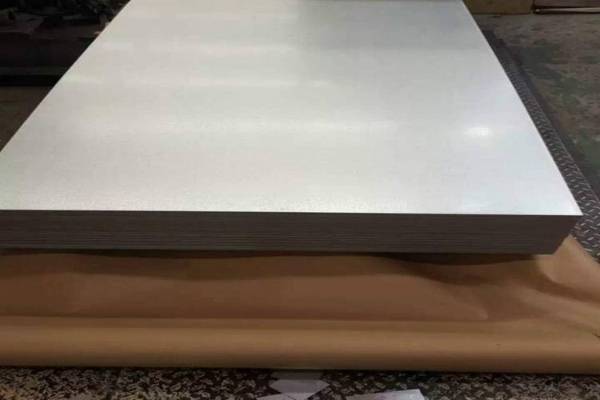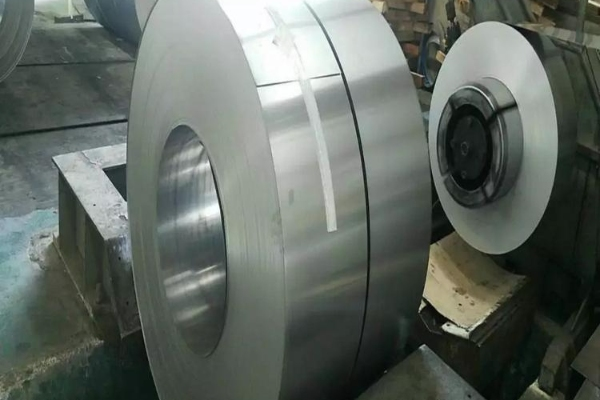Yield point elongation

The testing of mechanical properties is very important in the field of materials engineering. Among them, yield point and elongation are two key parameters used to assess the strength and plasticity of materials. In this paper, the definition, measurement methods and significance of yield point and elongation on material properties will be described in detail to help readers better understand these two concepts.

Definition and measurement of yield point

The yield point is the critical point where the material starts to deform in the process of force application, i.e., the point where the material starts to deform plastically and will not return to its initial state. Common yield point measurement methods include tensile and compression tests. Of these, the tensile test is the most commonly used method. The tensile test gradually increases the force on a material by applying a tensile force, recording the relationship between stress and strain. During the tensile process, the material goes through three stages: linear elasticity, plastic deformation and fracture. The yield point is usually between the linear elasticity and plastic deformation of the material. There are many methods of measuring the yield point, the most commonly used being the 0.2% deviation method. This method takes the point at which plastic deformation of the material begins to occur as the yield point, with a deviation of 0.2%. In addition, there are some other measurement methods, such as 1% deviation method, macro-plasticity method, etc., but 0.2% deviation method is widely accepted and applied in engineering practice.Meaning and application of yield point

Yield point is one of the important parameters to measure the strength of a material. In engineering design, knowing the yield point of a material helps in determining the maximum load that the material can withstand. In addition, the yield point can be used to determine the deformation limit of a material and thus assess the plasticity of the material. The yield point varies greatly from material to material, so it is critical for engineers to know the yield point when selecting a material.

Definition and measurement of elongation

Elongation is the degree to which a material can be extended after plastic deformation occurs during stress. Elongation is usually measured by elongation at break in a tensile test. Elongation at break is the difference between the length of a material before it breaks and the length after it breaks. Elongation at break is calculated by dividing the difference between the length before break and the length after break by the length before break and multiplying by 100%. High elongation means that the material has good plasticity and is capable of large plastic deformations during stress.

Meaning and application of elongation

Elongation is one of the important parameters for assessing the plasticity and toughness of a material. The elongation of a material determines the ability of the material to deform when subjected to a load. A high elongation means that the material has better ductility, can absorb more energy, and is more ductile when subjected to external forces. The elongation of a material is important for many engineering applications, such as the automotive, construction and aerospace industries. Choosing materials with high elongation improves the safety and reliability of your products.Conclusion

Yield point and elongation are two important indicators of the mechanical properties of a material. Yield point marks the beginning of plastic deformation of a material, while elongation evaluates how much a material deforms plastically during stress. Knowing and measuring these two parameters can help engineers select the right materials and design safer, more reliable products. In-depth research and understanding of testing methods for mechanical properties is vital to the development of the materials engineering field.

Related experiments